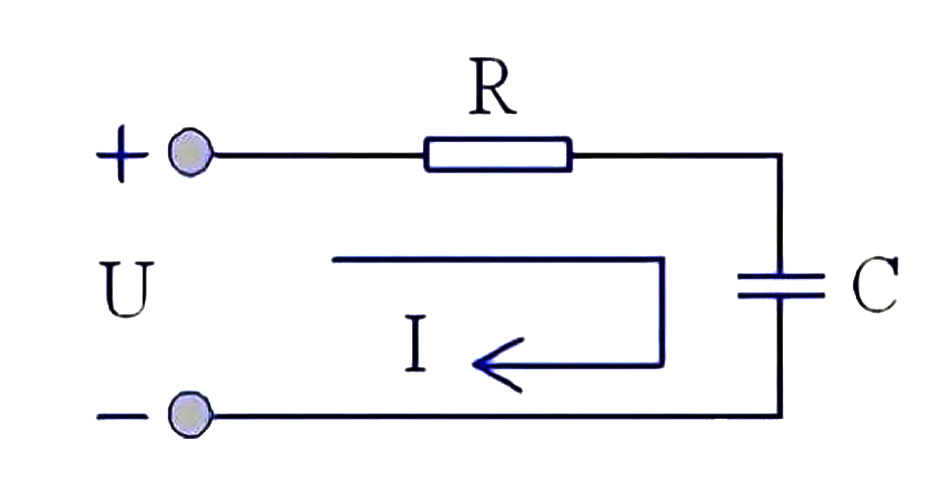1. Concept of resistance

As a component, resistance is also called resistor. The resistance of the conductor is usually represented by the letter R. The unit of resistance is ohm (ohm), abbreviated as OU, and the symbol is Ω (Greek letter, pronounced as Omega). Resistance belongs to an ordinary component, but has an unusual doorway, and the size of the resistance of the resistance component is generally related to temperature, material, length, and cross-sectional area, and the physical quantity to measure the size of the resistance affected by temperature is the temperature coefficient, which is defined as the percentage of the resistance value change when the temperature rises 1℃; Therefore, we generally pay attention to the value, precision, limit power, these three indicators can be appropriate.

2. The role of small resistance in series in the circuit

Resistors in series in the circuit is to make the current only one path, and resistors R1, R2 can be connected end to end, finally R1+R2 to get a total resistance value R.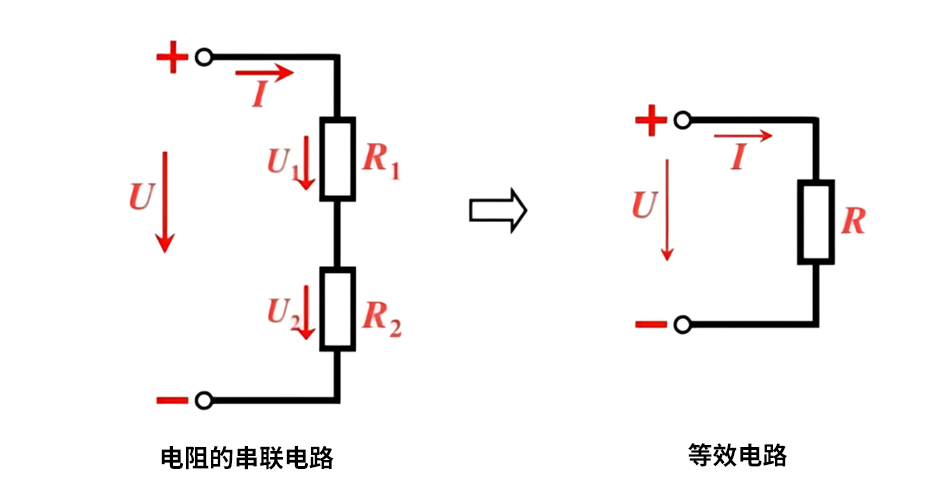However, resistance series in different circuits can play a role in a variety of, the following points are the role of resistance in the circuit:

1, partial pressure effect

No matter how big the resistance value is, it will play a role in the circuit in series. Because in a series circuit, the current across the resistance is equal, the sum of the voltages at each end of the resistance is equal to the total voltage of the circuit. It is known that the voltage on each resistor is less than the total voltage of the circuit, so the series resistance partial voltage. The formula in the figure below is the principle of partial pressure: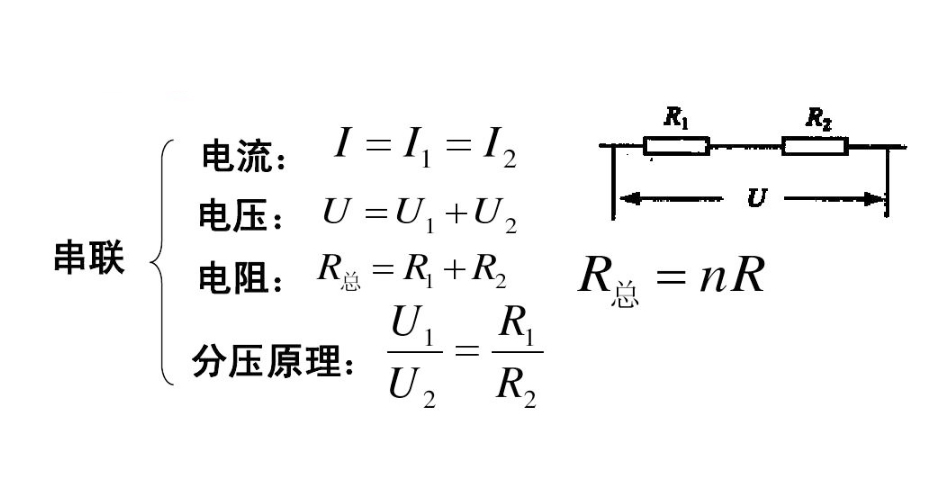2. Current limiting effect

In the circuit, some electronic components are “fragile”, if the current is too large, it will directly lead to the device burn; In this case, we usually use resistance in series in the circuit, so that the components do not exceed the normal range of values; In the following figure, the diode in the LED light-emitting circuit, if you want to make it work normally and not damaged, it needs to series a current-limiting resistor in which, and the selected resistance resistance value is determined according to the size of the components can withstand the current, so the resistance is small but also large resistance.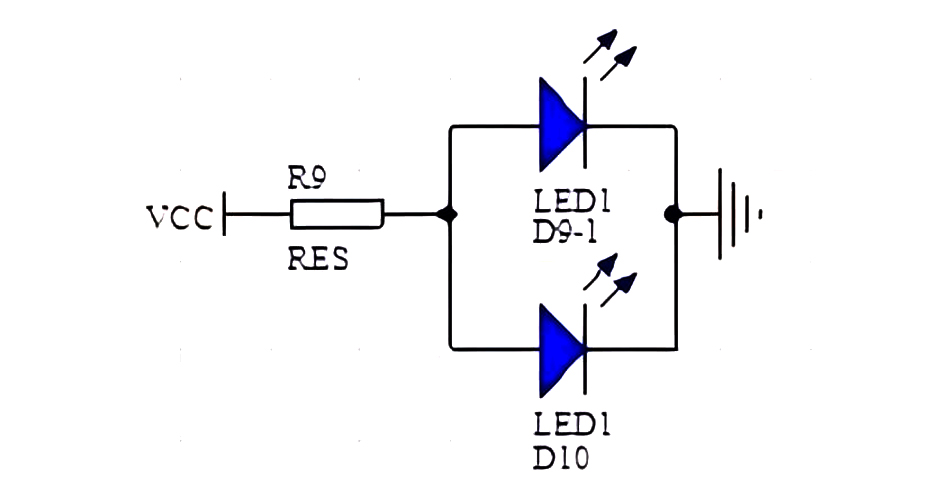3. The role of signal lines

The main purpose of small resistors in series in the signal line is to protect the pin, but its role can be divided into impedance matching and reducing the steepness of the signal edge. For example, the series resistors on the SPI signal line in the figure below are used to improve the matching, to reduce reflections, avoid oscillations, and facilitate debugging.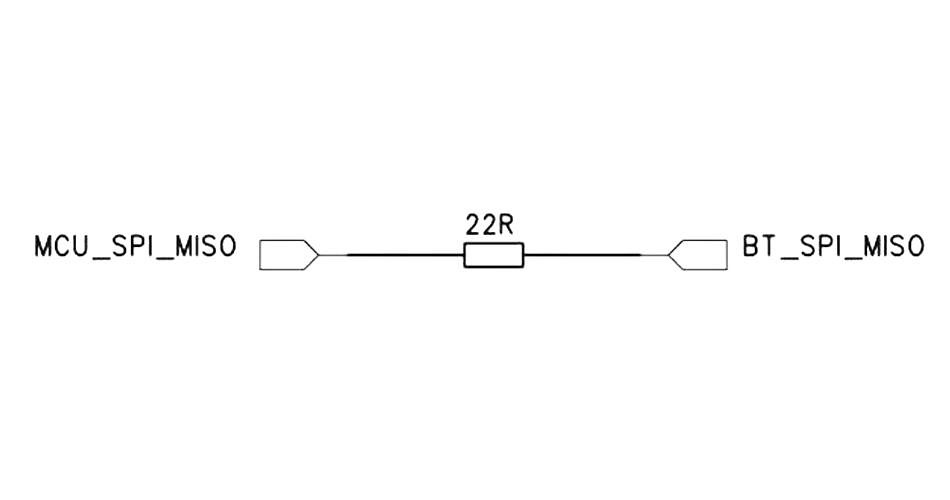4. The role of integral circuit

In the electronic circuit, one end of the capacitor is connected to the resistance, one end is grounded, filter the high frequency, equivalent to the integral, used for filtering, the most typical application is the amplifier circuit in the high and low audio control; For the power circuit, the resistance in series plays a damping role. The resistance consumes the energy of the overvoltage, thus suppressing the oscillation of the circuit.